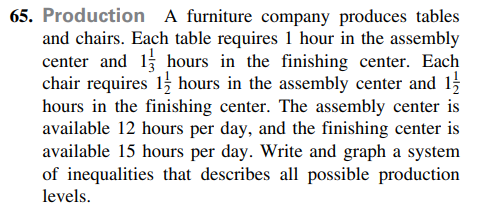### ¿Todavía tienes preguntas de matemáticas?

Pregunte a nuestros tutores expertos
Algebra
Pregunta

A furniture company produces tables and chairs. Each table requires $$1$$ hour in the assembly center and $$1 \frac { 1 } { 3 }$$ hours in the finishing center. Each chair requires $$1 \frac { 1 } { 2 }$$ hours in the assembly center and $$1 \frac { 1 } { 2 }$$

hours in the finishing center. The assembly center is available $$12$$ hours per day, and the finishing center is available $$15$$ hours per day. Write and graph a system of inequalities that describes all possible production levels.x + 3y/2 $$\le$$ 12
4x/3 + 3y/2 $$\le$$ 15